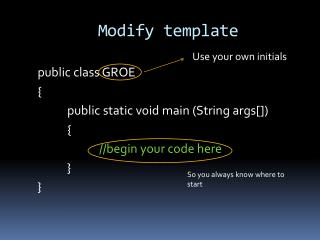DownloadDownload PresentationModify template

Modify template

Télécharger la présentationModify template

- - - - - - - - - - - - - - - - - - - - - - - - - - - E N D - - - - - - - - - - - - - - - - - - - - - - - - - - -
Presentation Transcript

1. Modify template Use your own initials public class GROE { public static void main (String args[]) { //begin your code here } } So you always know where to start

2. Resource book entry: reserved words Column A Column B • int declaration of an integer (whole number) • double declaration of a floating-point number (1.5, 10.333, PI, etc) • String declares a sequence of characters

3. Resource book entry: Special Commands Column A Column B + Addition operator. Also, used to separate strings from variables in System.out.print(); - Subtraction operator * Multiplication operator / Division operator % Modulus (remainder) operator = assigns left side to value on right side

4. Resource book entry: Vocabulary Column A Column B Typecasting converting from one data type to another. Ex. int x; double y; x = (int) y;

5. Practice program Use your own initials • www.compileonline.com/compile_java_online.php • Enter this code: public class GROEMath { public static void main(String args[]) { int x; int y; int sum; x = 10; //or another number of your choosing y = 15; //or another number of your choosing sum = x + y; //will not work without declarations of x, y, sum System.out.print(“The sum of ” + x + “ and “+ y +” is “ +sum); } } www.javalaunch.com

6. Save it • From now on, copy and paste your code to a notepad (or word) document. • Save as the same name as your class declaration with extension .java • Example: GROEMath.java

7. Your turn • Use the template, and write your code to do the following: • Declare variables x, y, sum, difference, product, (double) quotient, remainder. • Initialize x and y to the numbers 10 and 15 respectively • Have the program calculate the other variables and display them in a complete sentence (all 5) • Sentences should read similarly to: “The sum of 10 and 15 is 25” • Save and log out.